# How to change the negative numbers to zero in Excel?

The majority of employees who work with Excel spreadsheets are responsible for dealing with numbers included in either large or small datasets. When you work with numbers, you will encounter all varieties of them, including positive and negative numbers, decimals, date and time, and so on. The process of converting these numbers from one format to another is one of the more typical tasks that a lot of us need to accomplish on a regular basis.

When performing some computations, you may find that you need to convert negative numbers to positive numbers or to zero, that is removing the negative sign. This is probably the situation that arises the most frequently. If you are patient enough, you can certainly do it manually by going through each instance one at a time.

The majority of us are likely to consider sneaky tactics. Excel has a number of shortcuts that make it possible to convert negative numbers to zero quickly and easily.

In this tutorial, we are going to learn to change the negative number to zero through the following example.

## Using the IF Condition

The IF function is able to identify negative integers and reset them to zero without impacting the positive values it encounters. The IF function does a logical test, and it returns a True value if the test is successful, and it returns a False value if the test is unsuccessful. Let’s go through the following steps to convert negative numbers to zero using IF function

### Step1

In our example, we have some negative numbers along with some positive numbers in our Excel sheet.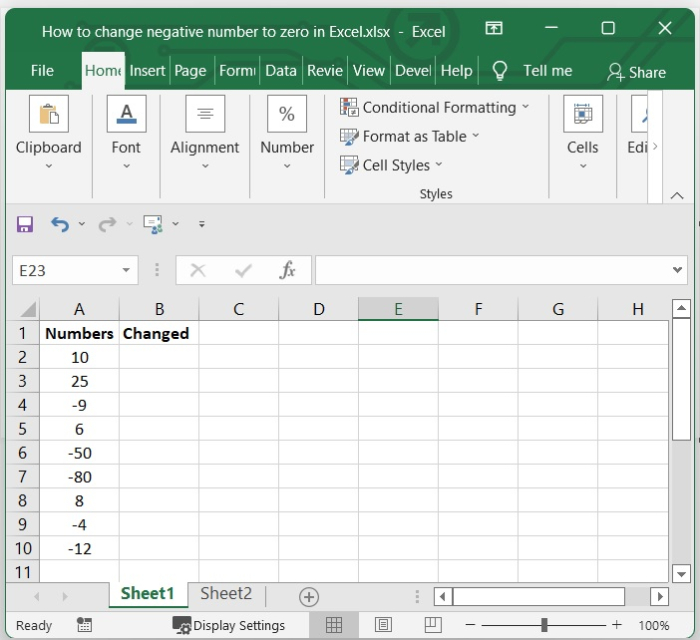### Step 2

Then in a cell which is empty, add the below given formula in formula bar and press enter.

In our example we have selected B2 and added the below given formula.

=IF(A2<0,0,A2)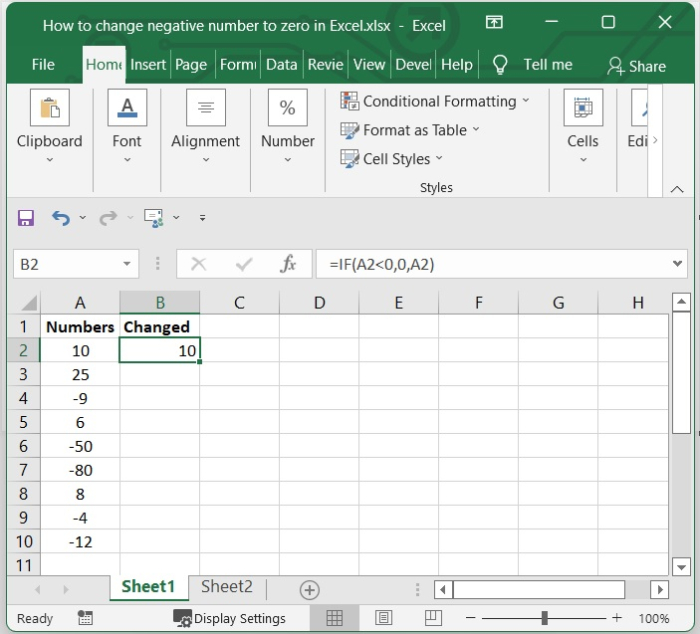### Step 3

You can select the range in which you want this formula to be applied by dragging the fill handle to the desired location. In addition to this, every negative integer has been replaced with a zero.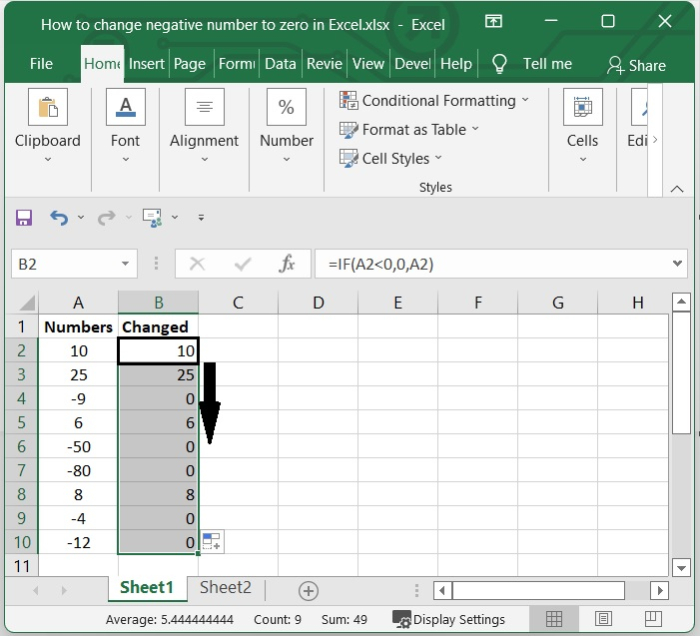## Using the MAX Condition

You can use a formula that is based on the MAX function to convert negative integers to zero without harming positive numbers. This can be accomplished by using the formula.

### Step 1

In our example, we have some negative numbers along with some positive numbers in our Excel sheet.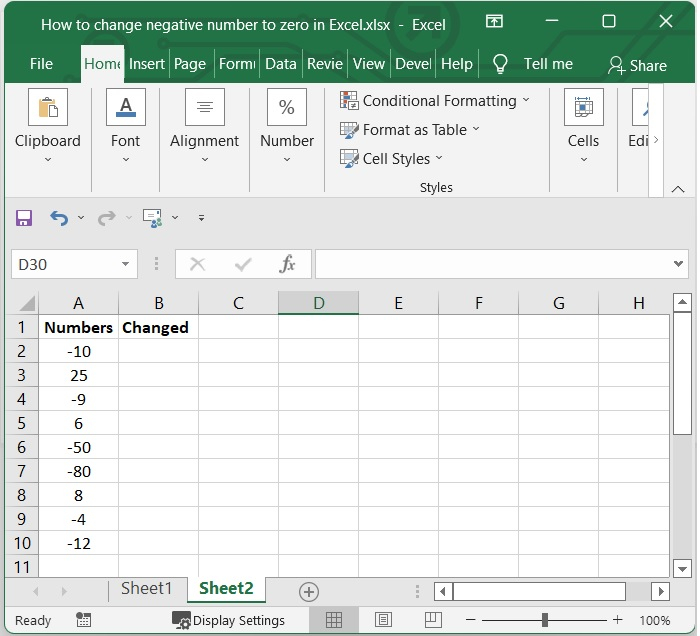### Step 2

Then Add the formula that is given below in the formula bar of a cell that is currently empty, and then hit enter.

In our example, we have selected B2 and added the below given formula.

=MAX(A2,0)


In our example, we apply formula for A2 cell. You can change as per your need. See the below given image.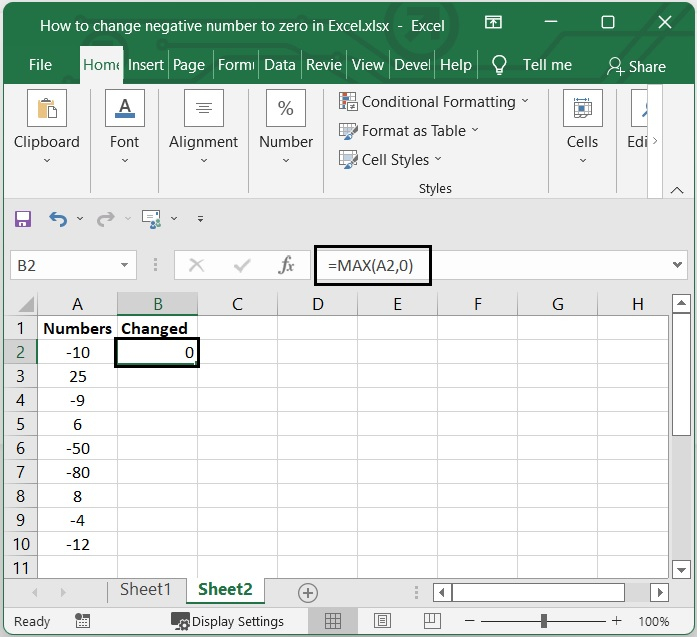### Step 3

By dragging the fill handle to the position where you would like the formula to be applied, you will have the option to choose the range that will be affected by the formula.

In addition to this, every negative integer has been changed to a zero throughout the entire calculation. See the following screenshot.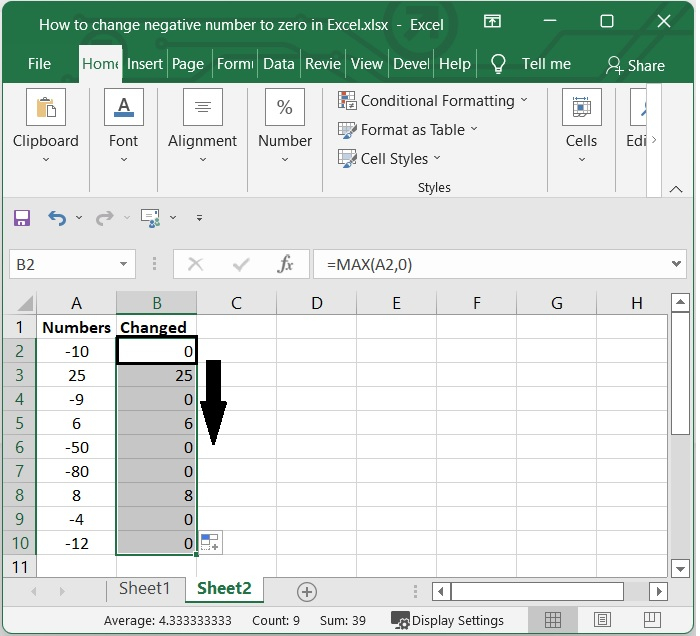## Conclusion

In this tutorial, we explained two methods (IF function and MAX function), using which you can convert negative numbers to zero in an Excel sheet.

Updated on: 06-Feb-2023

333 Views